# NCERT Solutions for Class 10 Maths Exercise 4.4## myCBSEguide App

CBSE, NCERT, JEE Main, NEET-UG, NDA, Exam Papers, Question Bank, NCERT Solutions, Exemplars, Revision Notes, Free Videos, MCQ Tests & more.

NCERT solutions for Maths Quadratic Equations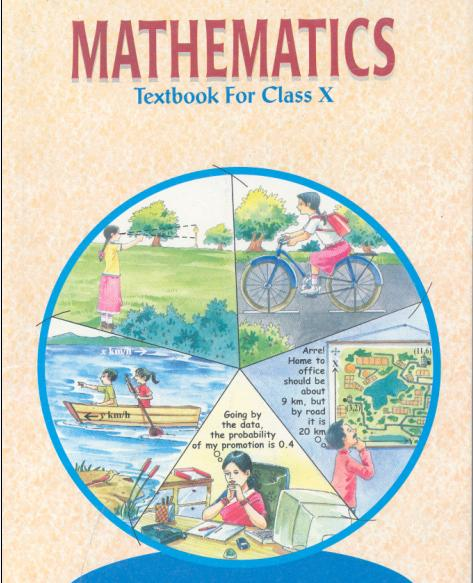## NCERT Solutions for Class 10 Maths Quadratic Equations

###### 1. Find the nature of the roots of the following quadratic equations. If the real roots exist, find them.

(i)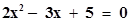(ii)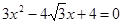(iii)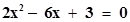Ans. (i)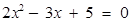Comparing this equation with general equation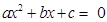,

We get a = 2, b = −3 and c = 5

Discriminant =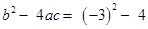(2) (5)

= 9 – 40 = −31

Discriminant is less than 0 which means equation has no real roots.

(ii)Comparing this equation with general equation,

We get a = 3, b =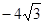and c = 4

Discriminant =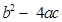=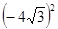− 4 (3) (4)

= 48 – 48 = 0

Discriminant is equal to zero which means equations has equal real roots.

Applying quadratic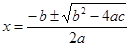to find roots,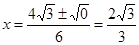Because, equation has two equal roots, it means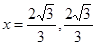(iii)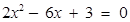Comparing equation with general equation,

We get a = 2, b = −6, and c = 3

Discriminant =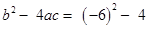(2) (3)

= 36 – 24 = 12

Value of discriminant is greater than zero.

Therefore, equation has distinct and real roots.

Applying quadratic formulato find roots,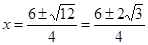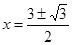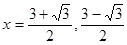NCERT Solutions for Class 10 Maths Exercise 4.4

###### 2. Find the value of k for each of the following quadratic equations, so that they have two equal roots.

(i)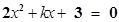(ii) kx (x − 2) + 6 = 0

Ans. (i)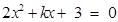We know that quadratic equation has two equal roots only when the value of discriminant is equal to zero.

Comparing equation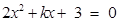with general quadratic equation, we get a = 2, b = k and c = 3

Discriminant =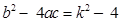(2) (3) =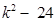Putting discriminant equal to zero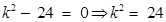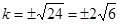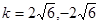(ii)kx (x − 2) + 6 = 0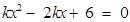Comparing quadratic equation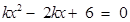with general form, we get a = k, b = −2k and c = 6

Discriminant =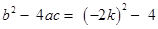(k) (6) =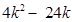We know that two roots of quadratic equation are equal only if discriminant is equal to zero.

Putting discriminant equal to zero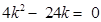⇒ 4k (k − 6) = 0⇒ k = 0, 6

The basic definition of quadratic equation says that quadratic equation is the equation of the form, where a ≠ 0.

Therefore, in equation, we cannot have k = 0.

Therefore, we discard k = 0.

Hence the answer is k = 6.

NCERT Solutions for Class 10 Maths Exercise 4.4

###### 3. Is it possible to design a rectangular mango grove whose length is twice its breadth, and the area is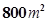. If so, find its length and breadth.

Ans. Let breadth of rectangular mango grove = x metres

Let length of rectangular mango grove = 2x metres

Area of rectangle =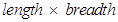=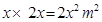According to given condition: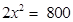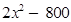= 0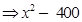= 0

Comparing equation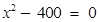with general form of quadratic equation, we get a = 1, b = 0 and c = −400

Discriminant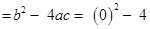(1) (−400) = 1600

Discriminant is greater than 0 means that equation has two disctinct real roots.

Therefore, it is possible to design a rectangular grove.

Applying quadratic formula,to solve equation,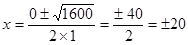x = 20, −20

We discard negative value of x because breadth of rectangle cannot be in negative.

Therefore, x = breadth of rectangle = 20 metres

Length of rectangle = 2x =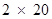= 40 metres

NCERT Solutions for Class 10 Maths Exercise 4.4

###### 4. Is the following situation possible? If so, determine their present ages.

The sum of the ages of two friends is 20 years. Four years ago, the product of their ages in years was 48.

Ans. Let age of first friend = x years and let age of second friend = (20 − x) years

Four years ago, age of first friend = (x − 4) years

Four years ago, age of second friend = (20 − x) − 4 = (16 − x) years

According to given condition,

(x − 4) (16 − x) = 48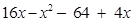= 48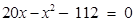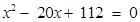Comparing equation,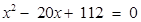with general quadratic equation, we get a = 1, b = −20 and c = 112

Discriminant =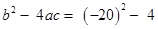(1) (112) = 400 – 448 = −48 < 0

Discriminant is less than zero which means we have no real roots for this equation.

Therefore, the give situation is not possible.

NCERT Solutions for Class 10 Maths Exercise 4.4

###### 5. Is it possible to design a rectangular park of perimeter 80 metres and area 400 m2. If so, find its length and breadth.

Ans. Let length of park = x metres

We are given area of rectangular park =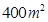Therefore, breadth of park =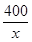metres{Area of rectangle = length × breadth}

Perimeter of rectangular park = 2 (length + breath) =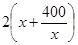metres

We are given perimeter of rectangle = 80 metres

According to condition: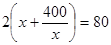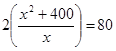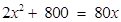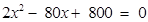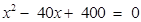Comparing equation,with general quadratic equation, we get a = 1, b = −40 and c = 400

Discriminant =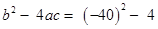(1) (400) = 1600 – 1600 = 0

Discriminant is equal to 0.

Therefore, two roots of equation are real and equal which means that it is possible to design a rectangular park of perimeter 80 metres and area.

Using quadratic formulato solve equation,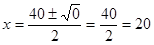Here, both the roots are equal to 20.

Therefore, length of rectangular park = 20 metres

Breadth of rectangular park =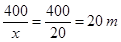## NCERT Solutions for Class 10 Maths Exercise 4.4

NCERT Solutions Class 10 Maths PDF (Download) Free from myCBSEguide app and myCBSEguide website. Ncert solution class 10 Maths includes text book solutions from Mathematics Book. NCERT Solutions for CBSE Class 10 Maths have total 15 chapters. 10 Maths NCERT Solutions in PDF for free Download on our website. Ncert Maths class 10 solutions PDF and Maths ncert class 10 PDF solutions with latest modifications and as per the latest CBSE syllabus are only available in myCBSEguide.

## CBSE app for Class 10

To download NCERT Solutions for Class 10 Maths, Computer Science, Home Science,Hindi ,English, Social Science do check myCBSEguide app or website. myCBSEguide provides sample papers with solution, test papers for chapter-wise practice, NCERT solutions, NCERT Exemplar solutions, quick revision notes for ready reference, CBSE guess papers and CBSE important question papers. Sample Paper all are made available through the best app for CBSE students and myCBSEguide website.### 14 thoughts on “NCERT Solutions for Class 10 Maths Exercise 4.4”

1. thank u

2. Thanku so much …u really helpinggg…

3. I found it as a best website ??

4. thank you so much ……i confused on this exercise thyan i enter on thiss app and i got all solutiuns

5. Thnxx ur solutions are vry helpful

6. Thank u so much these solution are to be helpful for us

7. THANKS IT’S HELPFUL TO ME .

8. This is really very supportive

9. Thanks u help me a lot

10. Thank you very much

11. Thank you

12. thankyou for this web because it is most helpful for us

13. thank u vfry much

14. Thank you so much